Professor Murray

Trigonometry in Right Angles

Slide Duration:

Section 1: Trigonometric Functions
Angles

39m 5s

Intro
0:00
Degrees
0:22
Circle is 360 Degrees
0:48
Splitting a Circle
1:13
2:08
2:31
2:52
Half-Circle and Right Angle
4:00
6:24
6:52
Coterminal, Complementary, Supplementary Angles
7:23
Coterminal Angles
7:30
Complementary Angles
9:40
Supplementary Angles
10:08
Example 1: Dividing a Circle
10:38
Example 2: Converting Between Degrees and Radians
11:56
Example 3: Quadrants and Coterminal Angles
14:18
Extra Example 1: Common Angle Conversions
-1
Extra Example 2: Quadrants and Coterminal Angles
-2
Sine and Cosine Functions

43m 16s

Intro
0:00
Sine and Cosine
0:15
Unit Circle
0:22
Coordinates on Unit Circle
1:03
Right Triangles
1:52
2:25
Master Right Triangle Formula: SOHCAHTOA
2:48
Odd Functions, Even Functions
4:40
Example: Odd Function
4:56
Example: Even Function
7:30
Example 1: Sine and Cosine
10:27
Example 2: Graphing Sine and Cosine Functions
14:39
Example 3: Right Triangle
21:40
Example 4: Odd, Even, or Neither
26:01
Extra Example 1: Right Triangle
-1
Extra Example 2: Graphing Sine and Cosine Functions
-2
Sine and Cosine Values of Special Angles

33m 5s

Intro
0:00
45-45-90 Triangle and 30-60-90 Triangle
0:08
45-45-90 Triangle
0:21
30-60-90 Triangle
2:06
Mnemonic: All Students Take Calculus (ASTC)
5:21
Using the Unit Circle
5:59
New Angles
6:21
9:43
Mnemonic: All Students Take Calculus
10:13
13:11
16:48
Example 3: All Angles and Quadrants
20:21
Extra Example 1: Convert, Quadrant, Sine/Cosine
-1
Extra Example 2: All Angles and Quadrants
-2
Modified Sine Waves: Asin(Bx+C)+D and Acos(Bx+C)+D

52m 3s

Intro
0:00
Amplitude and Period of a Sine Wave
0:38
Sine Wave Graph
0:58
Amplitude: Distance from Middle to Peak
1:18
Peak: Distance from Peak to Peak
2:41
Phase Shift and Vertical Shift
4:13
Phase Shift: Distance Shifted Horizontally
4:16
Vertical Shift: Distance Shifted Vertically
6:48
Example 1: Amplitude/Period/Phase and Vertical Shift
8:04
Example 2: Amplitude/Period/Phase and Vertical Shift
17:39
Example 3: Find Sine Wave Given Attributes
25:23
Extra Example 1: Amplitude/Period/Phase and Vertical Shift
-1
Extra Example 2: Find Cosine Wave Given Attributes
-2
Tangent and Cotangent Functions

36m 4s

Intro
0:00
Tangent and Cotangent Definitions
0:21
Tangent Definition
0:25
Cotangent Definition
0:47
Master Formula: SOHCAHTOA
1:01
Mnemonic
1:16
Tangent and Cotangent Values
2:29
Remember Common Values of Sine and Cosine
2:46
90 Degrees Undefined
4:36
Slope and Menmonic: ASTC
5:47
Uses of Tangent
5:54
Example: Tangent of Angle is Slope
6:09
7:49
Example 1: Graph Tangent and Cotangent Functions
10:42
Example 2: Tangent and Cotangent of Angles
16:09
Example 3: Odd, Even, or Neither
18:56
Extra Example 1: Tangent and Cotangent of Angles
-1
Extra Example 2: Tangent and Cotangent of Angles
-2
Secant and Cosecant Functions

27m 18s

Intro
0:00
Secant and Cosecant Definitions
0:17
Secant Definition
0:18
Cosecant Definition
0:33
Example 1: Graph Secant Function
0:48
Example 2: Values of Secant and Cosecant
6:49
Example 3: Odd, Even, or Neither
12:49
Extra Example 1: Graph of Cosecant Function
-1
Extra Example 2: Values of Secant and Cosecant
-2
Inverse Trigonometric Functions

32m 58s

Intro
0:00
Arcsine Function
0:24
Restrictions between -1 and 1
0:43
Arcsine Notation
1:26
Arccosine Function
3:07
Restrictions between -1 and 1
3:36
Cosine Notation
3:53
Arctangent Function
4:30
Between -Pi/2 and Pi/2
4:44
Tangent Notation
5:02
Example 1: Domain/Range/Graph of Arcsine
5:45
Example 2: Arcsin/Arccos/Arctan Values
10:46
Example 3: Domain/Range/Graph of Arctangent
17:14
Extra Example 1: Domain/Range/Graph of Arccosine
-1
Extra Example 2: Arcsin/Arccos/Arctan Values
-2
Computations of Inverse Trigonometric Functions

31m 8s

Intro
0:00
Inverse Trigonometric Function Domains and Ranges
0:31
Arcsine
0:41
Arccosine
1:14
Arctangent
1:41
Example 1: Arcsines of Common Values
2:44
Example 2: Odd, Even, or Neither
5:57
Example 3: Arccosines of Common Values
12:24
Extra Example 1: Arctangents of Common Values
-1
Extra Example 2: Arcsin/Arccos/Arctan Values
-2
Section 2: Trigonometric Identities
Pythagorean Identity

19m 11s

Intro
0:00
Pythagorean Identity
0:17
Pythagorean Triangle
0:27
Pythagorean Identity
0:45
Example 1: Use Pythagorean Theorem to Prove Pythagorean Identity
1:14
Example 2: Find Angle Given Cosine and Quadrant
4:18
Example 3: Verify Trigonometric Identity
8:00
Extra Example 1: Use Pythagorean Identity to Prove Pythagorean Theorem
-1
Extra Example 2: Find Angle Given Cosine and Quadrant
-2
Identity Tan(squared)x+1=Sec(squared)x

23m 16s

Intro
0:00
Main Formulas
0:19
Companion to Pythagorean Identity
0:27
For Cotangents and Cosecants
0:52
How to Remember
0:58
Example 1: Prove the Identity
1:40
Example 2: Given Tan Find Sec
3:42
Example 3: Prove the Identity
7:45
Extra Example 1: Prove the Identity
-1
Extra Example 2: Given Sec Find Tan
-2

52m 52s

Intro
0:00
0:09
How to Remember
0:48
Cofunction Identities
1:31
How to Remember Graphically
1:44
Where to Use Cofunction Identities
2:52
Example 1: Derive the Formula for cos(A-B)
3:08
Example 2: Use Addition and Subtraction Formulas
16:03
Example 3: Use Addition and Subtraction Formulas to Prove Identity
25:11
Extra Example 1: Use cos(A-B) and Cofunction Identities
-1
Extra Example 2: Convert to Radians and use Formulas
-2
Double Angle Formulas

29m 5s

Intro
0:00
Main Formula
0:07
How to Remember from Addition Formula
0:18
Two Other Forms
1:35
Example 1: Find Sine and Cosine of Angle using Double Angle
3:16
Example 2: Prove Trigonometric Identity using Double Angle
9:37
Example 3: Use Addition and Subtraction Formulas
12:38
Extra Example 1: Find Sine and Cosine of Angle using Double Angle
-1
Extra Example 2: Prove Trigonometric Identity using Double Angle
-2
Half-Angle Formulas

43m 55s

Intro
0:00
Main Formulas
0:09
Confusing Part
0:34
Example 1: Find Sine and Cosine of Angle using Half-Angle
0:54
Example 2: Prove Trigonometric Identity using Half-Angle
11:51
Example 3: Prove the Half-Angle Formula for Tangents
18:39
Extra Example 1: Find Sine and Cosine of Angle using Half-Angle
-1
Extra Example 2: Prove Trigonometric Identity using Half-Angle
-2
Section 3: Applications of Trigonometry
Trigonometry in Right Angles

25m 43s

Intro
0:00
Master Formula for Right Angles
0:11
SOHCAHTOA
0:15
Only for Right Triangles
1:26
Example 1: Find All Angles in a Triangle
2:19
Example 2: Find Lengths of All Sides of Triangle
7:39
Example 3: Find All Angles in a Triangle
11:00
Extra Example 1: Find All Angles in a Triangle
-1
Extra Example 2: Find Lengths of All Sides of Triangle
-2
Law of Sines

56m 40s

Intro
0:00
Law of Sines Formula
0:18
SOHCAHTOA
0:27
Any Triangle
0:59
Graphical Representation
1:25
Solving Triangle Completely
2:37
When to Use Law of Sines
2:55
ASA, SAA, SSA, AAA
2:59
SAS, SSS for Law of Cosines
7:11
Example 1: How Many Triangles Satisfy Conditions, Solve Completely
8:44
Example 2: How Many Triangles Satisfy Conditions, Solve Completely
15:30
Example 3: How Many Triangles Satisfy Conditions, Solve Completely
28:32
Extra Example 1: How Many Triangles Satisfy Conditions, Solve Completely
-1
Extra Example 2: How Many Triangles Satisfy Conditions, Solve Completely
-2
Law of Cosines

49m 5s

Intro
0:00
Law of Cosines Formula
0:23
Graphical Representation
0:34
Relates Sides to Angles
1:00
Any Triangle
1:20
Generalization of Pythagorean Theorem
1:32
When to Use Law of Cosines
2:26
SAS, SSS
2:30
Heron's Formula
4:49
Semiperimeter S
5:11
Example 1: How Many Triangles Satisfy Conditions, Solve Completely
5:53
Example 2: How Many Triangles Satisfy Conditions, Solve Completely
15:19
Example 3: Find Area of a Triangle Given All Side Lengths
26:33
Extra Example 1: How Many Triangles Satisfy Conditions, Solve Completely
-1
Extra Example 2: Length of Third Side and Area of Triangle
-2
Finding the Area of a Triangle

27m 37s

Intro
0:00
Master Right Triangle Formula and Law of Cosines
0:19
SOHCAHTOA
0:27
Law of Cosines
1:23
Heron's Formula
2:22
Semiperimeter S
2:37
Example 1: Area of Triangle with Two Sides and One Angle
3:12
Example 2: Area of Triangle with Three Sides
6:11
Example 3: Area of Triangle with Three Sides, No Heron's Formula
8:50
Extra Example 1: Area of Triangle with Two Sides and One Angle
-1
Extra Example 2: Area of Triangle with Two Sides and One Angle
-2
Word Problems and Applications of Trigonometry

34m 25s

Intro
0:00
Formulas to Remember
0:11
SOHCAHTOA
0:15
Law of Sines
0:55
Law of Cosines
1:48
Heron's Formula
2:46
Example 1: Telephone Pole Height
4:01
Example 2: Bridge Length
7:48
Example 3: Area of Triangular Field
14:20
Extra Example 1: Kite Height
-1
Extra Example 2: Roads to a Town
-2
Vectors

46m 42s

Intro
0:00
Vector Formulas and Concepts
0:12
Vectors as Arrows
0:28
Magnitude
0:38
Direction
0:50
Drawing Vectors
1:16
Uses of Vectors: Velocity, Force
1:37
Vector Magnitude Formula
3:15
Vector Direction Formula
3:28
Vector Components
6:27
Example 1: Magnitude and Direction of Vector
8:00
Example 2: Force to a Box on a Ramp
12:25
Example 3: Plane with Wind
18:30
Extra Example 1: Components of a Vector
-1
Extra Example 2: Ship with a Current
-2
Section 4: Complex Numbers and Polar Coordinates
Polar Coordinates

1h 7m 35s

Intro
0:00
Polar Coordinates vs Rectangular/Cartesian Coordinates
0:12
Rectangular Coordinates, Cartesian Coordinates
0:23
Polar Coordinates
0:59
Converting Between Polar and Rectangular Coordinates
2:06
R
2:16
Theta
2:48
Example 1: Convert Rectangular to Polar Coordinates
6:53
Example 2: Convert Polar to Rectangular Coordinates
17:28
Example 3: Graph the Polar Equation
28:00
Extra Example 1: Convert Polar to Rectangular Coordinates
-1
Extra Example 2: Graph the Polar Equation
-2
Complex Numbers

35m 59s

Intro
0:00
Main Definition
0:07
Number i
0:23
Complex Number Form
0:33
Powers of Imaginary Number i
1:00
Repeating Pattern
1:43
Operations on Complex Numbers
3:30
3:39
Multiplying Complex Numbers
4:39
FOIL Method
5:06
Conjugation
6:29
Dividing Complex Numbers
7:34
Conjugate of Denominator
7:45
Example 1: Solve For Complex Number z
11:02
Example 2: Expand and Simplify
15:34
Example 3: Simplify the Powers of i
17:50
Extra Example 1: Simplify
-1
Extra Example 2: All Complex Numbers Satisfying Equation
-2
Polar Form of Complex Numbers

40m 43s

Intro
0:00
Polar Coordinates
0:49
Rectangular Form
0:52
Polar Form
1:25
R and Theta
1:51
Polar Form Conversion
2:27
R and Theta
2:35
Optimal Values
4:05
Euler's Formula
4:25
Multiplying Two Complex Numbers in Polar Form
6:10
Multiply r's Together and Add Exponents
6:32
Example 1: Convert Rectangular to Polar Form
7:17
Example 2: Convert Polar to Rectangular Form
13:49
Example 3: Multiply Two Complex Numbers
17:28
Extra Example 1: Convert Between Rectangular and Polar Forms
-1
Extra Example 2: Simplify Expression to Polar Form
-2
DeMoivre's Theorem

57m 37s

Intro
0:00
Introduction to DeMoivre's Theorem
0:10
n nth Roots
3:06
DeMoivre's Theorem: Finding nth Roots
3:52
Relation to Unit Circle
6:29
One nth Root for Each Value of k
7:11
Example 1: Convert to Polar Form and Use DeMoivre's Theorem
8:24
Example 2: Find Complex Eighth Roots
15:27
Example 3: Find Complex Roots
27:49
Extra Example 1: Convert to Polar Form and Use DeMoivre's Theorem
-1
Extra Example 2: Find Complex Fourth Roots
-2
Bookmark & Share Embed

## Copy & Paste this embed code into your website’s HTML

Please ensure that your website editor is in text mode when you paste the code.
(In Wordpress, the mode button is on the top right corner.)
×
• - Allow users to view the embedded video in full-size.
Since this lesson is not free, only the preview will appear on your website.

• ## Related Books1 answerLast reply by: Dr. Will MurraySun Oct 4, 2020 12:02 PMPost by Hong Yang on October 3, 2020thanks sir, this course helped me out a lot1 answerLast reply by: Dr. Will MurrayMon Jul 20, 2020 11:29 AMPost by Erika Wu on July 18, 2020on number three example trying to figure out "fee" you want need to use the soh  part of sohcahtoa since you know 180 - 90- 62.8 is 21.81 answerLast reply by: Dr. William MurrayWed Oct 14, 2015 4:44 PMPost by Vance Bower on October 14, 2015Can you use sin, cosin, or tangent in any order for angle theta or c to get the angle measurements? In other words, you used the tan of theta and the sin of c in the third example. Could I have used the tangent of theta and the cosign or tangent of c, instead of the sin of c?2 answersLast reply by: Dr. William MurrayTue Aug 5, 2014 11:50 AMPost by Joshua Jacob on July 19, 2014Sorry if this is a little unrelated but is it possible to do arcsin, arccos, and arctan on a graphing calculator? Is it the same thing as sin^-1...etc. Thank you for making these amazing lectures!1 answerLast reply by: Dr. William MurrayThu Jul 18, 2013 8:25 AMPost by Manfred Berger on July 7, 2013Unless I'm missing anything crucial here, the fact that SOCAHTOA only works for right triangles stems directly from the basic definition of the the unit circle, which is essentially the pythagorian theorem.1 answerLast reply by: Dr. William MurrayMon May 6, 2013 8:54 PMPost by Emily Engle on May 5, 2013How would you solve for the inverse ratios without a calculator?

### Trigonometry in Right Angles

Main formulas:

Master formula for right triangles: SOHCAHTOA!

 sinθ = opposite sidehypotenuse cosθ = adjacent sidehypotenuse tanθ = opposite sideadjacent side

Example 1:

A right triangle has short sides of length 3 and 4. Find all the angles in the triangle.

Example 2:

A right triangle has one angle measuring 40°  and opposite side of length 6. Find the lengths of all the sides.

Example 3:

The lengths of the two short sides of a right triangle are in a 5:2 ratio. Find all angles of the triangle.

Example 4:

A right triangle has short sides of length 3 and hypotenuse of length 7. Find all the angles in the triangle.

Example 5:

A right triangle has one angle of 65°  and hypotenuse of length 3. Find the lengths of all the sides of the triangle.

### Trigonometry in Right Angles

A right triangle has short sides of length 5 and 12. Find all the angles in the triangle
• Start by drawing a right triangle with labeled side lengths and angles
•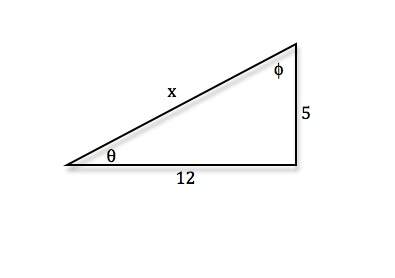• Recall SOHCAHTOA, sin x = [Opposite/Hypotenuse], cos x = [Adjacent/Hypotenuse], tan x = [Opposite/Adjacent]
• tanθ = [5/12] ⇒θ = arctan([5/12]) ⇒θ ≈ 22.6° (Make sure your calculator is in degree mode)
• tanϕ = [12/5] ⇒ϕ = arctan([12/5]) ⇒ϕ ≈ 67.4°
A right triangle has angle measure 33° and adjacent length 7. Find the missing angle and the lengths of the other two sides of the triangle
• Start by drawing a right triangle with labeled side lengths and angles
•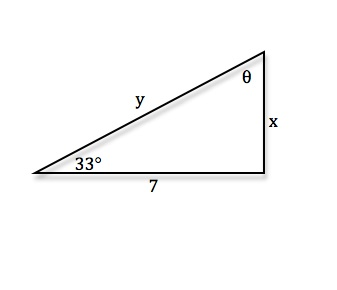• Recall SOHCAHTOA, sin x = [Opposite/Hypotenuse], cos x = [Adjacent/Hypotenuse], tan x = [Opposite/Adjacent]
Missing angle: θ = 180° − 90° − 33° ⇒θ = 57°

sin33° = [x/7] ⇒ x = 7sin(33°) ⇒ x ≈ 3.8 (Make sure your calculator is in degree mode

cos33° = [7/y] ⇒ y = [7/(cos33°)] ⇒ y ≈ 8.3
The lengths of two short sides of a right triangle are in a 7:4 ratio. Find the missing side length and all the angles of the triangle
• Start by drawing a right triangle with labeled side lengths and angles
•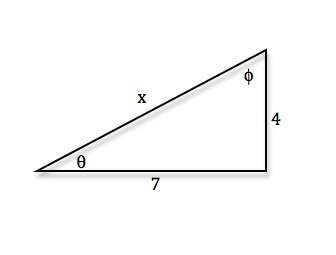• Recall SOHCAHTOA, sin x = [Opposite/Hypotenuse], cos x = [Adjacent/Hypotenuse], tan x = [Opposite/Adjacent]
• Missing side length: Use Pythagorean Theorem a2 + b2 = c2
Missing side length: x2 = 72 + 42 ⇒ x2 = 49 + 16 ⇒ x2 = 65 ⇒ x = √{65}

tanθ = [7/4] ⇒θ = arctan([7/4]) ⇒θ≈ 60.3°

sinϕ = [4/(√{65} )] ⇒ϕ = arcsin([4/(√{65} )]) ⇒ ϕ ≈ 29.7°
A right triangle has short side of length 4 and hypotenuse of length 9. Find the missing side length and all the angles of the triangle
• Start by drawing a right triangle with labeled side lengths and angles
•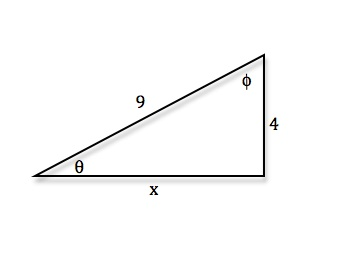• Recall SOHCAHTOA, sin x = [Opposite/Hypotenuse], cos x = [Adjacent/Hypotenuse], tan x = [Opposite/Adjacent]
• Missing side length: Use Pythagorean Theorem a2 + b2 = c2
Missing side length: 92 = x2 + 42 ⇒ 81 = x2 + 16 ⇒ x2 = 65 ⇒ x = √{65}

sinθ = [4/9] ⇒θ = arcsin([4/9]) ⇒θ ≈ 26.4°

cosϕ = [4/9] ⇒ϕ = arccos([4/9]) ⇒ ϕ ≈ 63.6°
A right triangle has one angle of 56° and hypotenuse of length 7. Find the lengths of all the sides and the missing angle
• Start by drawing a right triangle with labeled side lengths and angles
•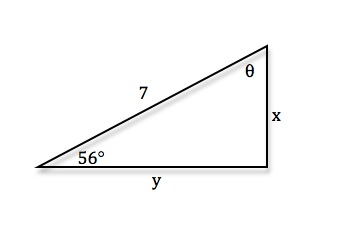• Recall SOHCAHTOA, sin x = [Opposite/Hypotenuse], cos x = [Adjacent/Hypotenuse], tan x = [Opposite/Adjacent]
Missing angle: θ = 180° − 90° − 56° ⇒θ = 34°

sin56° = [x/7] ⇒ x = 7sin56° ⇒ x ≈ 5.8

cos56° = [y/7] ⇒ y = 7cos56° ⇒ y ≈ 3.9
A right triangle has short sides of length 11 and 12. Find all the angles in the triangle
• Start by drawing a right triangle with labeled side lengths and angles
•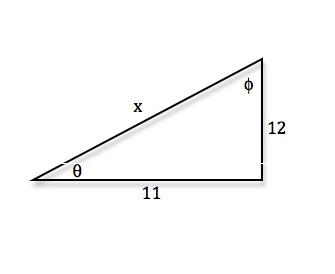• Recall SOHCAHTOA, sin x = [Opposite/Hypotenuse], cos x = [Adjacent/Hypotenuse], tan x = [Opposite/Adjacent]
• tanθ = [12/11] ⇒θ = arctan([12/11]) ⇒ θ ≈ 47.5° (Make sure your calculator is in degree mode)
• tanϕ = [11/12] ⇒ϕ = arctan([11/12]) ⇒ϕ ≈ 42.5°
A right triangle has angle measure 27° and opposite side length 4. Find the missing angle and the lengths of the other two sides of the triangle
• Start by drawing a right triangle with labeled side lengths and angles
•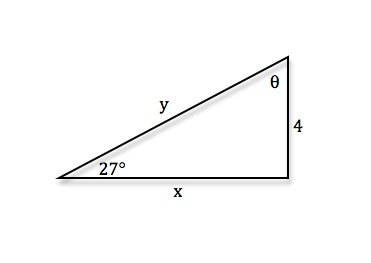• Recall SOHCAHTOA, sin x = [Opposite/Hypotenuse], cos x = [Adjacent/Hypotenuse], tan x = [Opposite/Adjacent]
Missing angle: θ = 180° - 90° - 27° ⇒θ = 57°

sin27° = [4/y] ⇒ y = [4/(sin27°)] ⇒ y ≈ 8.8 (Make sure your calculator is in degree mode)

tan27° = [4/x] ⇒ x = [4/(tan27°)] ⇒ x ≈ 7.9
The lengths of two short sides of a right triangle are in a 6:3 ratio. Find the missing side length and all the angles of the triangle
• Start by drawing a right triangle with labeled side lengths and angles
•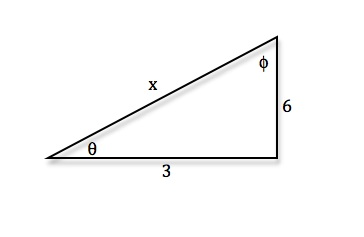• Recall SOHCAHTOA, sin x = [Opposite/Hypotenuse], cos x = [Adjacent/Hypotenuse], tan x = [Opposite/Adjacent]
• Missing side length: Use Pythagorean Theorem a2 + b2 = c2
Missing side length: x2 = 32 + 62 ⇒ x2 = 9 + 36 ⇒ x2 = 45 ⇒ x = √{45}

tanθ = [6/3] ⇒θ = arctan([6/3]) ⇒θ ≈ 63.4°

tanϕ = [3/6] ⇒ϕ = arctan([3/6]) ⇒ϕ ≈ 26.6°
A right triangle has short side of length 7 and hypotenuse of length 11. Find the missing side length and all the angles of the triangle
• Start by drawing a right triangle with labeled side lengths and angles
•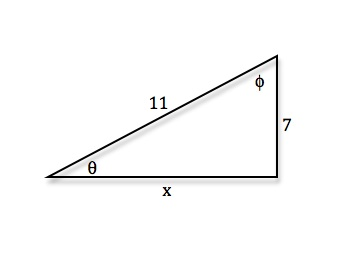• Recall SOHCAHTOA, sin x = [Opposite/Hypotenuse], cos x = [Adjacent/Hypotenuse], tan x = [Opposite/Adjacent]
• Missing side length: Use Pythagorean Theorem a2 + b2 = c2
Missing side length: 112 = x2 + 72 ⇒ 121 = x2 + 49 ⇒ x2 = 72 ⇒ x = 6√2

sinθ = [7/11] ⇒θ = arcsin([7/11]) ⇒θ ≈ 39.5°

cosϕ = [7/11] ⇒ϕ = arccos([7/11]) ⇒ϕ ≈ 50.5°
A right triangle has one angle of 68° and hypotenuse of length 9. Find the lengths of all the sides and the missing angle
• Start by drawing a right triangle with labeled side lengths and angles
•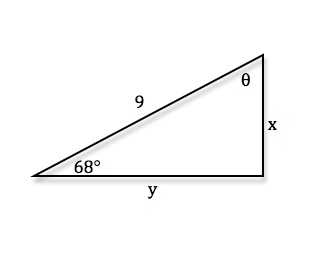• Recall SOHCAHTOA, sin x = [Opposite/Hypotenuse], cos x = [Adjacent/Hypotenuse], tan x = [Opposite/Adjacent]
Missing angle: θ = 180° − 90° − 68° ⇒θ = 22°

sin68° = [x/9] ⇒ x = 9sin68° ⇒ x ≈ 8.3

cos68° = [y/9] ⇒ y = 9cos68° ⇒ y ≈ 3.4

*These practice questions are only helpful when you work on them offline on a piece of paper and then use the solution steps function to check your answer.

### Trigonometry in Right Angles

Lecture Slides are screen-captured images of important points in the lecture. Students can download and print out these lecture slide images to do practice problems as well as take notes while watching the lecture.

• Intro 0:00
• Master Formula for Right Angles 0:11
• SOHCAHTOA
• Only for Right Triangles
• Example 1: Find All Angles in a Triangle 2:19
• Example 2: Find Lengths of All Sides of Triangle 7:39
• Example 3: Find All Angles in a Triangle 11:00
• Extra Example 1: Find All Angles in a Triangle
• Extra Example 2: Find Lengths of All Sides of Triangle

### Transcription: Trigonometry in Right Angles

We are trying some examples of right angle in trigonometry, we are finding lengths of sides and angles in right triangles.0000

The master formula we are using here is SOHCAHTOA, and remember that SOHCAHTOA only works in triangles where one of the angles is a right angle, so in right triangles.0012

SOHCAHTOA does not work in other kind of triangles, we are going to learn some complicated rules later on called the law of cosines and the law of sines, that will work in any kind of triangle.0026

Right now we are just looking at right triangles and we are using SOHCAHTOA to figure out the relationships between the length of the sides and the measure of the angles.0037

What we are given here is a right triangle with a short side of length = 3 and hypotenuse of length = 7.0046

We want to find all the angles in the triangle so let me try to graph this out.0056

We know that it is a right triangle, one angle is a right angle, short side has length = 3 and the hypotenuse has length = 7, we want to find all the angles in the triangle.0067

I label the angles as theta and phi, I think it will be probably useful to find the third side of the triangle.0079

we know that the third side satisfies if I call it x for the time being.0091

x2 + 32 = 72, so x2 + 9 = 49.0096

X2 is 40 and x = square root of 40, we can factor 4 out of that so 2 square root of 10.0108

That one did not come out to be very neat as some of other triangles have then.0121

I want to find this angles now, theta if I use SOHCAHTOA, I know that sin (theta) is equal to the opposite / hypotenuse.0125

That one I deliberately picked one where I would not have to use the square root to make my life a little bit simpler.0137

So, opposite is 3, the hypotenuse is 7, theta itself is arcsin(3/7).0142

Now remember here, if you calculator is set to radian mode then you will get a very strange answer looking here.0154

If you are looking for degrees, you have to set your calculator to degree mode.0161

On my calculator that is a matter of pushing the mode button and then moving it over and selecting the degree option.0166

It has two options, degree and radians, you want to set the degree options if that is the kind of answer you are looking for.0172

In my case, I’m going to do the inverse sin of 3 / 7, and it tells me that it is 25.4 degrees.0180

That tells me what theta is, I would like to figure out now what phi is, if I use the sin = opposite / hypotenuse.0202

If I use that for phi then I’m going to have to look at this ugly number 2 square root of 10.0211

Instead, I’m going to use cos(phi) = adjacent/ hypotenuse, phi is right there so the adjacent is 3.0218

Cos(pi) is 3/7, phi is arcos(3/7) and if I work that out on my calculator I get 64.6 degrees approximately.0230

I’m rounding here to the nearest decimal place, so now I have phi.0252

That gives me all the angles of the triangle because the last angle is a right angle, but I want to check these by seeing whether these angles adds up to 180 degrees.0258

25.4 + 64.6 those add up to 90 and the last angle is a 90 degree angle, it does in fact give me a 180 degrees.0270

That is very satisfying to see that does checked, the key there was to remember the SOHCAHTOA formula which works in all right triangles.0286

And then when we figured out that we are given two sides of the triangle, we are able to figure out the angles using sin(theta) =( opposite / hypotenuse), cos(phi) = adjacent / hypotenuse.0294

For our last example of right triangle trigonometry, we are given a right triangle that has one angle of 65 degrees and a hypotenuse of length=3.0000

I want to find the lengths of all the sides of the triangle, let me draw this out.0012

We got one angle on the corner, I will call it (theta) and we are given that 65 degrees, we got a right angle and we know that the hypotenuse is length = 3.0022

The question is to find the lengths of the other sides of the triangle and I’m going to use my standard formula SOHCAHTOA, (Some Old Horse Caught Another Horse Taking Oats Away).0033

I’m going to use the sin portion of that formula, so sin(theta) = (opposite/hypotenuse).0048

I do not know what the opposite is but I know that the hypotenuse is 3 and I know that (theta) is 65 degrees.0064

The opposite is equal to 3 x sin(65 degrees), remember to set your calculator to degree mode.0076

If you have it set in radian you will get a very confusing looking answer here.0083

I typed in 3 × sin(65) and I get that, that is approximately equal to 2.7.0090

That tells me that the side opposite theta is approximately 2.7 units long.0100

Finally, I am going to use the cos part of SOHCAHTOA, cos(theta) = adjacent/hypotenuse, and the hypotenuse is still 3 units, the cos(theta) is cos(65 degrees).0107

If we solve that for the adjacent side, we get ((3 cos(65 degrees)) and the calculator tells me that it is approximately equal 1.3 units.0129

That tells me that the adjacent side to (theta) is 1.3.0137

Now again, I figured out each one of those sides using SOHCAHTOA, I’m going to check it using the Pythagorean theorem.0159

As I check here, I will do 1.32 + 2.72 and that should be approximately equal to 1.32 + 2.72.0168

If you work that out on the calculator that actually gives you 8.98.0187

There is a little bit of rounding when I found those values that is very close to 9 which is 3 squared.0192

That tells us that the Pythagorean theorem is satisfied by these lengths which means that we almost certainly did those right.0200

Again, that came back to writing down all the information we had in the triangle and labeling one of the angles.0206

We labeled the angle we were given as (theta=65 degrees) and then using the SOHCAHTOA relationships to set up some equations.0212

And then solving for the length of the sides that we did not know, the sin gave us the length of the opposite side.0221

The cos SOHCAHTOA relationship gave us the length of the adjacent side.0230

And then it was an easy matter to check that those actually satisfy the Pythagorean theorem.0235

That is the end of the lecture on trigonometry in right triangles.0240

We will come back later and talk about trigonometry in triangles that do not necessarily have a right angle.0245

We will be using the law of sines and law of cosines to analyze those.0251

Thanks for watching the trigonometry lectures on www.educator.com.0255

Hi, these are the trigonometry lectures for educator.com.0000

Today, we're going to talk about trigonometry in triangles that have a right angle.0005

These are called right triangles.0009

The master formula for right triangles, we've seen it before it's the SOH CAH TOA.0011

That's the word that I remember to know that the sin(θ) ...0017

Let me draw a right triangle here.0022

If θ is one of the small angles, not the right angle, then the sin(θ) is equal to the length of the opposite side over the length of the hypotenuse.0026

Cos(θ) is equal to the adjacent side over the hypotenuse.0042

The tan(θ) is equal to the opposite side over the adjacent side.0050

For shorthand, sine is equal to opposite over hypotenuse, cosine is equal to adjacent over hypotenuse, tangent is equal to opposite over adjacent.0053

It's probably worth saying SOH CAH TOA often enough until it sticks into your memory, because it really is useful for remembering these things.0063

If you have a hard time remembering that, the little mnemonic that is also helpful for some students is Some Old Horse Caught Another Horse Taking Oats Away.0071

That spells out SOH CAH TOA for you.0083

One key thing to remember here is that SOH CAH TOA only works in right triangles.0085

You have to have one angle being a right angle.0093

If you have a triangle that is not a right triangle, if you don't have a right angle, don't use SOH CAH TOA because it's not valid in triangles that don't have right angles.0096

That's if you have no right angle.0116

We're going to learn in the next lectures on educator.com, we'll learn about the law of sines and the law of cosines.0122

Those work in any triangle where you don't need a right angle, but when you have a right angle, it's definitely easier and better and quicker to use SOH CAH TOA.0128

Let's try that out with some actual triangles.0138

On the first example, we have a right triangle with short sides of length 3 and 4, and we want to find all the angles in the triangle.0140

Let me draw a triangle here.0149

We're told that the short sides have length 3 and 4.0154

Of course, one angle is a right angle, so I don't need to worry about that.0158

I'll call these angles θ, and I'll call this one φ.0162

First thing we're going to need to know is what the hypotenuse of this triangle is.0166

h2=32+42, Pythagorean theorem there, which is 9+16, which is 25.0172

So, h is the square root of 25, h is 5.0184

Let me draw that in there.0187

Now, I want to figure out what θ and φ are.0191

I'm going to use SOH CAH TOA.0197

Let me write that down there for reference, SOH CAH TOA.0200

I'm going to figure out what θ is by using the SOH part of the SOH CAH TOA.0205

Sin(θ) is equal to the opposite over the hypotenuse.0214

The opposite angle to θ is 3 and the hypotenuse is 5.0225

So, θ=arcsin(3/5).0231

I'm going to work that out on the calculator.0238

My calculator has an arcsine button, it actually writes it as sin-1, which I don't like that notation because makes it seem like a power.0241

In any case, I'm going to use inverse sine of 3/5.0251

There's a very important step here that many students get confused about which is that, if you're looking for an answer in terms of degrees, which in real world measurement, it sometimes easier to use degrees than radians.0257

You have to set your calculator to degree mode.0270

Most calculators have a degree mode and a radian mode.0274

In fact, all calculators that do trigonometric functions have a degree mode and a radian mode.0278

The default is probably radian mode.0284

If your calculator is set in radian mode and you try to do something like arcsin(3/5), you'll get an answer that doesn't look right.0287

You may be confused if you're checking your answers somewhere, it may not agree with what the correct answer it.0297

What you have to do is set up your calculator in degree mode, if you want an answer in degrees.0304

My calculator is a Texas Instruments.0311

It's got a mode button, I just scroll down, it say's RAD and DEG to convert it from radians to degrees.0315

I'm going to convert it into degree mode and then I'll get an answer in terms of degrees.0323

That's a step that many students forget and they get kind of confused when they get an answer which is in terms of radians, but it doesn't agree with the degree answer they were looking for.0330

Now, I've got my calculator set in degree mode.0338

I'll do the arcsin(3/5) which is 0.6.0342

It tells me that that is approximately equal to 36.9 degrees.0348

I found one of the angles in the triangle.0358

Of course, another one is a right angle, so it's 90 degrees.0359

For φ, I think for φ, I'm going to practice the cosine part of the SOH CAH TOA.0362

I know that cos(φ) is equal to adjacent over hypotenuse.0369

That's the adjacent side to φ, so φ is over here, it's adjacent side is 3, hypotenuse is still 5.0378

So, φ=arccos(3/5).0390

Again, I'll do that on my calculator.0396

The calculator tells me that that's 53.1 degrees, approximately equal to 53.1 degrees.0404

Now, there's a little check here you can do to make sure that you work this out correctly.0412

We know that the angles of a triangle add up to 180 degrees.0416

If we check here, 36.9+53.1 plus the last angle was a 90-degree angle, a right angle, if you add those up, 36.9+53.1 is 90 plus 90, you get 180 degrees.0421

That tells me that my answers are right.0439

The key formula here to remember is SOH CAH TOA.0443

Everything comes down to drawing the angles in the triangle, and just using sine equals opposite over hypotenuse, cosine equals adjacent over hypotenuse, tangent equals opposite over adjacent.0446

Now, we're given a right triangle and we're told that one angle measures 40 degrees.0460

Let me call that angle right here, that would be 40 degrees.0472

The opposite side has length 6.0480

I want to find the lengths of all the sides in the triangle.0486

Of course, finding the angles is no big deal because one side is a right angle, we're told that it is a right triangle.0491

I can find the other angle just by subtracting from 180.0498

In fact, 180-40 is 140, minus 90, is 50.0502

I know that other angle is 50.0511

The challenge here is to find the lengths.0513

We're going to use SOH CAH TOA.0516

I'm going to apply SOH CAH TOA to 40.0521

I know that sin(40) is equal to 6 over the hypotenuse, because that's opposite over hypotenuse.0526

The hypotenuse, if I solve this, that's equal to 6/sin(40).0535

Remember to convert your calculator to degree mode before you do this kind of calculation.0545

6/sin(40) is approximately equal to 9.3.0551

That tells us the length of the hypotenuse, 9.3.0561

Now I'd like to find the length of the other sides.0567

I'm going to use the cosine part of SOH CAH TOA.0572

I know that cos(40) is equal to the adjacent over the hypotenuse.0574

If I solve that for the adjacent side, that's equal to hypotenuse times the cos(40).0585

I know the hypotenuse now.0592

If I multiply that by cos(40).0596

What I get is approximately 7.2 for my adjacent side.0601

The sides of my triangle are 6, 7.2 and 9.3.0611

Again, there's an easy way to check that.0618

We'll check that using the Pythagorean theorem.0620

I want to check that 62+7.22 gives me 87.8, which is approximately equal to 9.32.0623

I know that I got those side lengths right.0656

The third example here, we have the lengths of the two short sides of a right triangle, are in a 5:2 ratio.0662

Let me draw that out.0668

We're given that it's a right triangle.0676

We've got lengths in a 5:2 ratio.0679

I don't actually know that these lengths are 5 and 2, but here's the thing, if I expand this triangle proportionately, it won't change the angles.0683

If I blow this up to a similar triangle that actually has side lengths of 5 and 2, I'll get a similar triangle with the same angles.0695

I can just assume that this triangle has actually side lengths of 5 and 2.0706

I want to find all the angles in the triangle.0711

I'll label that one as θ, that one is φ.0715

I know that sooner or later, I'm going to need the hypotenuse of the triangle, I'll go ahead and find that now.0719

h2=22+52, that's equal to 4+25 which is 29.0722

My hypotenuse is the square root of 29.0738

I'd like to practice all parts of SOH CAH TOA, and we've used sine and cosine in the previous problems.0747

I'm going to use the tangent part.0757

Remember, tangent is opposite over adjacent.0760

Tan(θ) there, the opposite side is 5, and the adjacent side has length 2.0765

I'm going to find arctan(5/2), θ is arctan(5/2).0772

Remember that you want to have your calculator in degree mode here, because if you have your calculator in radian mode, you'll get an answer in radian which would look very different from any answer in degrees that you were expecting.0783

I calculate arctan(5/2), and it tells me that that is approximately equal to 68.2 degrees.0800

Now, I found θ, I've got to find φ now.0815

I could find φ just by subtracting θ from 90 degrees, because I know θ+φ adds to 90 degrees.0819

Remember, one angle of the triangle is already 90 degrees, the other two must add up to 90 degrees.0826

I could just find the other angle by subtracting but I want to avoid that.0833

I want to practice using my SOH CAH TOA rules.0839

The other reason is if I find it using some other method, then I can add them together at the end and use that to check my work.0841

I'm going to try using a SOH CAH TOA rule.0848

I'm going to find it using angle φ.0853

Looking at φ, I know that sin(φ) is equal to the opposite over hypotenuse.0854

Sin(φ) is equal to the opposite over hypotenuse.0866

The opposite side to φ is 2, and the hypotenuse is the square root of 29.0872

φ is equal to the arcsine of 2 over the square root of 29.0881

That's definitely something I want to put into my calculator.0886

I'll figure out inverse sine of 2 divided by square root of 29 ...0891

The inverse sine of 2 divided by square root of 29 ...0911

It tells me that that's approximately equal to 21.8 degrees.0917

That's what I got using SOH CAH TOA.0925

Again, I'm going to check it by checking that the three angles of this triangle add up to a 180 degrees.0928

I've got 68.2 degrees plus 21.8 degrees, those add up to 90, plus the last 90-degree angle, the right angle.0937

Those do add up to 180 degrees.0951

That tells me that my work must probably be right.0954

That came back to looking at SOH CAH TOA, and figuring out what the angles were based on the SOH CAH TOA.0958

We know that tangent is opposite over adjacent, and we also used that sine is opposite over hypotenuse.0968

We'll try some more examples later.0974

OR

### Start Learning Now

Our free lessons will get you started (Adobe Flash® required).